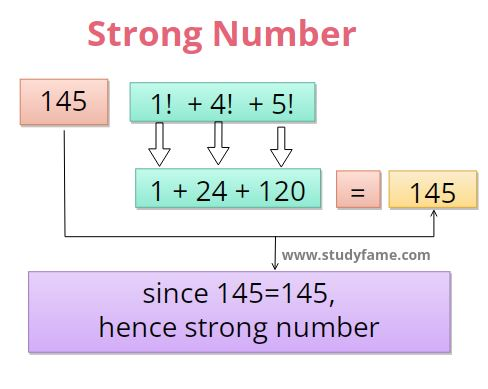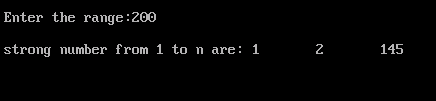# Write C program to find a Strong number

When the sum of the factorial of each individual digit is equal to an original number then a number is said to be a strong number. For example 145=1! + 4! + 5! are Strong numbers.

List of Strong number are: 1, 2, 145, 40585, ..## C program to check strong number

In this program, you will write a C program to check whether the number entered by the user is a Strong number or not.

``````
#include <stdio.h>
int main()
{
int remainders,fact,sum=0,i,num,copynum;
printf("Enter the number:");
scanf("%d",&num);
copynum=num;
while(num!=0)
{
remainders=num%10;
fact=1;
for(i=1;i<=remainders;i++)
{
fact=fact*i;
}
sum=sum+fact;
num=num/10;
}
if(sum==copynum)
{
printf("%d is strong numbers",copynum);
}
else
{
printf("%d is not a strong numbers",copynum);
}
return 0;
}
``````

## output

```Enter the number:145
145 is strong numbers```

## Check Strong Number explanation

1. In this program, you will take a number from the user and store it in variable `num`.
2. copy the value of `num` into another variable `copynum` for verification purposes i.e `copynum=num`.
3. Inside the loop find the last digit of the given number `num` and stored the last digit value in `remainders` variables i.e `remainder=num%10`.
4. find the factorial of `remainders` value and store it in variable `fact`.
``````for(i=1;i<=remainders;i++)
{
fact=fact*i;
}``````
5. add the factorial value in the variable sum. `sum=sum+fact`
6. divide num by 10. so that it removes the last digit from the `num` variable as it is not needed anymore. `num=num/10`
7. you will repeat steps 3 to 6 until `num` is 0.
8. after the end, you will check whether `copynum`  is the same as the `sum` value i.e `sum==copynum`. if it is the same, then the number is strong otherwise it is not a strong number.

## Program to find strong number from 1 to n

``````
#include <stdio.h>
int main()
{
int remainders,sum,fact,range,i,num,n;
printf("\n Enter the range:");
scanf("%d",&range);
printf("\n strong number from 1 to n are:");
for(n=1;n<=range;n++)
{
sum=0;
num=n;
while(num>0)
{
remainders=num%10;
fact=1;
for(i=1;i<=remainders;i++)
{
fact=fact*i;
}
sum=sum+fact;
num=num/10;
}
if(sum==n)
{
printf("\t%d",n);
}
}
return 0;
}
``````

## output## Program to find strong number in a given range

``````#include <stdio.h>
int main()
{
int remainders,sum,fact,lower,upper,i,num,n;
printf("Enter the lower range:");
scanf("%d",&lower);
printf("Enter the upper range:");
scanf("%d",&upper);
printf("strong number from %d to %d are:",lower,upper);
for(n=lower;n<=upper;n++)
{
sum=0;
num=n;
while(num>0)
{
remainders=num%10;
fact=1;
for(i=1;i<=remainders;i++)
{
fact=fact*i;
}
sum=sum+fact;
num=num/10;
}
if(sum==n)
{
printf("\t%d",n);
}
}
return 0;
}
``````

## output

```Enter the lower range:2
Enter the upper range:400
strong number from 2 to 400 are: 2       145```

## Related Programs

Prime number program in C using for loop, while loop
palindrome program in C
Armstrong number program in C